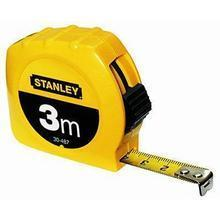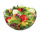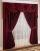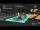# Conversion of units

Complete the following length data

Correct result:

6m 2dm =  6.2 m
8cm 3mm =  8.3 cm
5km 86m =  5.086 km

#### Solution:We would be pleased if you find an error in the word problem, spelling mistakes, or inaccuracies and send it to us. Thank you!Tips to related online calculators
Need help calculate sum, simplify or multiply fractions? Try our fraction calculator.
Do you want to convert length units?

## Next similar math problems:

• Math homeworkIt took Jose two-thirds of an hour to complete his math homework on Monday, three-fourths of an hour on Tuesday, any two- fifths of an hour on Wednesday. How many hours did it take Jose to complete his homework altogether?
• MilimetersHow many millimeters is 1/4 meters?We need two tenths kg of a carrot, one tenth of peas and three tenths of of tomatoes to make salad. Express the fraction of the weight of the vegetables to be salad. Convert the result to grams.
• Clock mathematicsIf it is now 7:38 pm, what time will it be in 30,033,996,480 minutes from now?
• Interior designerTo make draperies an interior designer needs 11 1/4 yards of material for the den and 8 1/2 yards for the living room. If material comes only in 20 yard bolts, how much will be left over after completing both sets of draperies?
• DayWhat part of the day is 23 hours 22 minutes? Express as a decimal number.Add and write the result again as hours, minutes, seconds: 2hodiny45min15s + 1h20m50s =
• ExpressionsLet k represent an unknown number, express the following expressions: 1. The sum of the number n and two 2. The quotient of the number n and nine 3. Twice the number n 4. The difference between nine and the number n 5. Nine less than the number n
• Length subtractingExpress in mm: 5 3/10 cm - 2/5 mm
• TimeWrite time in minutes rounded to one decimal place: 5 h 28 m 26 s.
• The thirdThe one-third rod is blue, one-half of the rod is red, the rest of the rod is white and measures 8 cm. How long is the whole rod?
• ChestnutsThree divisions of nature protectors participated in the collection of chestnut trees.1. the division harvested 1250 kg, the 2nd division by a fifth more than the 1st division and the 3rd division by a sixth more than the second division. How many tons ofThe voltage station is every day changing the master password, which consists of three letters. Code generation process does not change and is based on the following procedure: The following letters (A) to (I) correspond to different numbers from 1 to 9.How many steps of 76 cm circumvent square garden with area 1.8 ha?2 and 1 8th plus 1 and 1 3rd =The two brothers were to be divided according to the will of land at an area of 1ha 86a 30m2 in a ratio of 5:4. How many will everyone get?Add this two mixed numbers: 1 5/6 + 2 2/11=